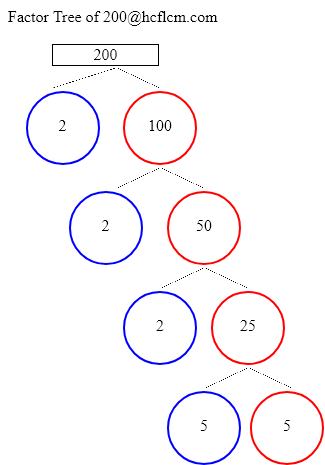# Factors of 200 | Find the Factors of 200 by Factoring Calculator

Factoring Calculator calculates the factors and factor pairs of positive integers.

Factors of 200 can be calculated quickly with the help of Factoring Calculator i.e. 1, 2, 4, 5, 8, 10, 20, 25, 40, 50, 100, 200 positive integers that divide 200 without a remainder.

Factors of 200 are 1, 2, 4, 5, 8, 10, 20, 25, 40, 50, 100, 200. There are 12 integers that are factors of 200. The biggest factor of 200 is 200.

Factors of:

### Factor Tree of 200 to Calculate the Factors

 200 2 100 2 50 2 25 5 5Factors of 200 are 1, 2, 4, 5, 8, 10, 20, 25, 40, 50, 100, 200. There are 12 integers that are factors of 200. The biggest factor of 200 is 200.

Positive integers that divides 200 without a remainder are listed below.

• 1
• 2
• 4
• 5
• 8
• 10
• 20
• 25
• 40
• 50
• 100
• 200

### Factors of 200 in pairs

• 1 × 200 = 200
• 2 × 100 = 200
• 4 × 50 = 200
• 5 × 40 = 200
• 8 × 25 = 200
• 10 × 20 = 200
• 20 × 10 = 200
• 25 × 8 = 200
• 40 × 5 = 200
• 50 × 4 = 200
• 100 × 2 = 200
• 200 × 1 = 200

### Factors of 200 Table

FactorFactor Number
1one
2two
4four
5five
8eight
10ten
20twenty
25twenty five
40forty
50fifty
100one hundred
200two hundred

### How to find Factors of 200?

As we know factors of 200 are all the numbers that can exactly divide the number 200 simply divide 200 by all the numbers up to 200 to see the ones that result in zero remainders. Numbers that divide without remainder are factors and in this case below are the factors

1, 2, 4, 5, 8, 10, 20, 25, 40, 50, 100, 200 are the factors and all of them can exactly divide number 200.

### Frequently Asked Questions on Factors of 200

1. What are the factors of 200?

Answer: Factors of 200 are the numbers that leave a remainder zero. The ones that can divide 200 exactly i.e. factors are 1, 2, 4, 5, 8, 10, 20, 25, 40, 50, 100, 200.

2.What are Factor Pairs of 200?

Answer:Factor Pairs of 200 are

• 1 × 200 = 200
• 2 × 100 = 200
• 4 × 50 = 200
• 5 × 40 = 200
• 8 × 25 = 200
• 10 × 20 = 200
• 20 × 10 = 200
• 25 × 8 = 200
• 40 × 5 = 200
• 50 × 4 = 200
• 100 × 2 = 200
• 200 × 1 = 200

3. What is meant by Factor Pairs?

Answer:Factor Pairs are numbers that when multiplied together will result in a given product.